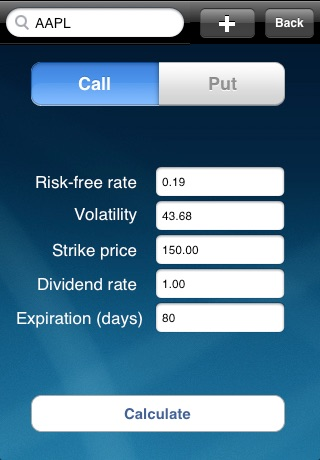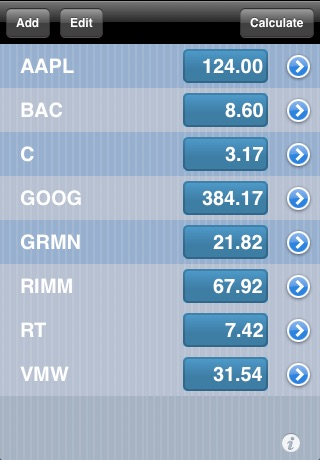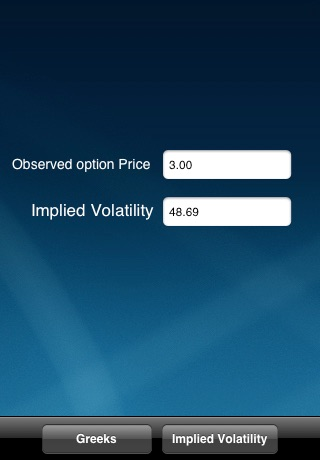Version: 1.00
Size: 0.3 MB
Free

## Description

```This application uses the Black-Scholes model to calculate the price of an option (put/call) related to any underlying security traded in the U.S. markets. The inputs used in this calculation are the strike price, the price of the underlying security, the risk-free rate, time to maturity, the dividend rate, and the volatility of the underlying security.  We have provided close approximations to the price of the underlying security, the risk-free rate, and the volatility. The application automatically updates these inputs based on time sensitivity but the user is not restricted to them, each user can change any input to any figure he/she feels comfortable with. The other inputs (strike price, dividend rate, and time to maturity) aren’t provided automatically and are to be inputted at the user’s discretion. In using this inputs, the main output that is calculated is the price of the option (call/put), but we have also provided other outputs known as ‘The Greeks’ that represent the sensitivity of the option to changes in any of the input variables. Such Greeks include; the delta, gamma, vega, theta and rho.

The second part of the application calculates the implied volatility of the option (cal/put), this is the volatility implied by the market price of the option based on the Black-Scholes model. In other words, it is the volatility that, when used in the Black-Scholes model, yields a theoretical value for the option equal to the current market price of that option. Implied volatility is known as a forward-looking measure, that differs from the historical volatility (which is calculated based on past movements in the price of the security).```

## Screenshots for для Айфона, Айпада